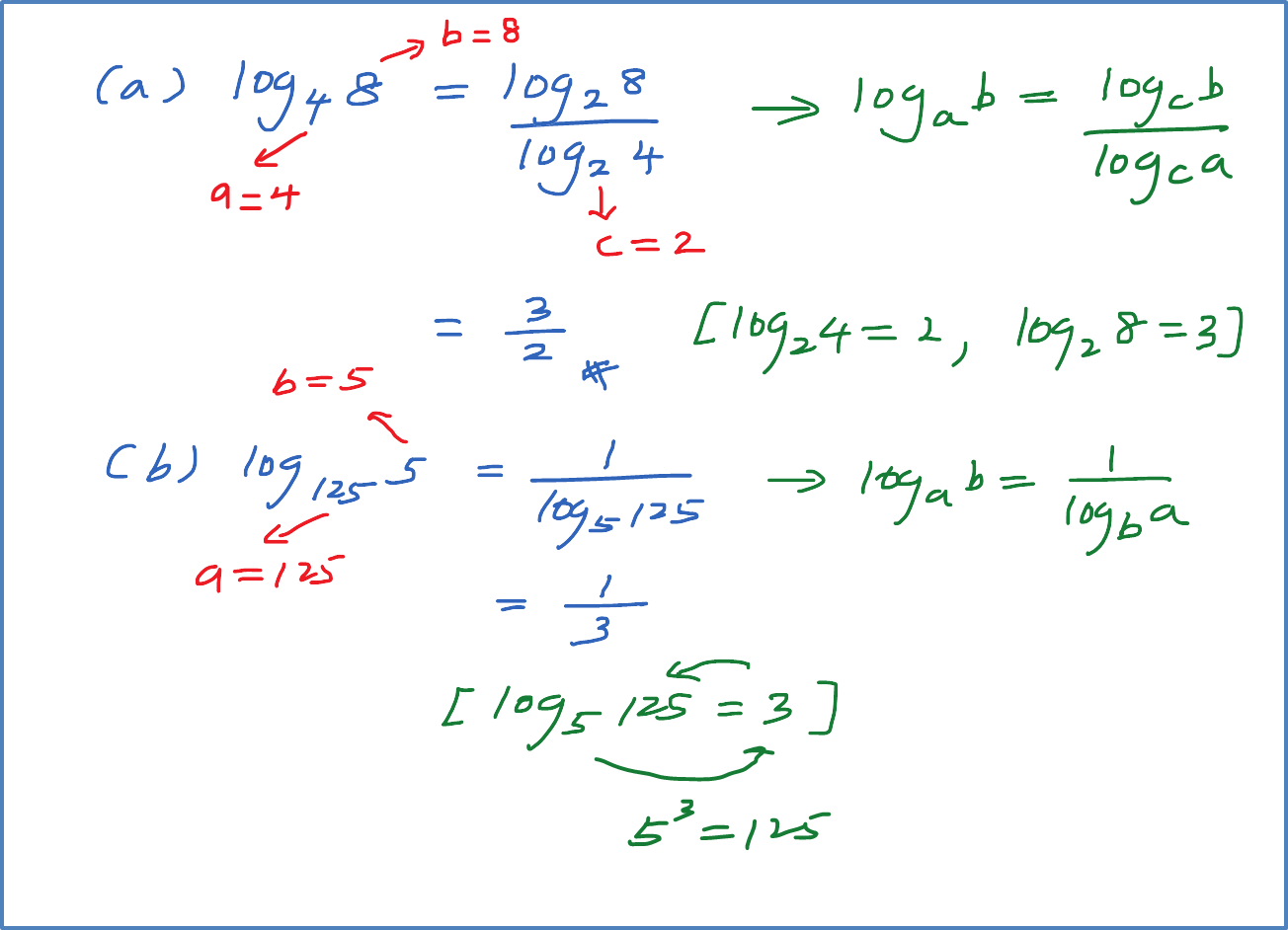\

# 4.2.2 Change of Base of Logarithms

### Change of Base of Logarithms

Example
:
Find the value of the following:
a. ${\mathrm{log}}_{25}100$
b. ${\mathrm{log}}_{3}0.45$

Example 1
Find the value of the following.
(a) ${\mathrm{log}}_{4}8$
(b) ${\mathrm{log}}_{125}5$
(c) ${\mathrm{log}}_{81}27$
(d) ${\mathrm{log}}_{16}64$Example 2
Given that ${\mathrm{log}}_{p}3=h$   and ${\mathrm{log}}_{p}5=k$  , express the following in term of h and or k.
(a) ${\mathrm{log}}_{p}\frac{5}{3}$

(b) ${\mathrm{log}}_{15}75$### 1 thought on “4.2.2 Change of Base of Logarithms”

1.a. log 100 should be log 10 to the power of 2, equal 2